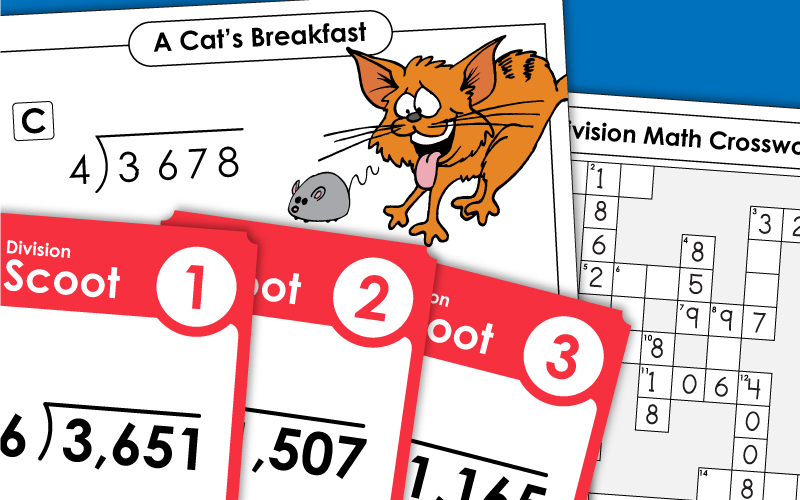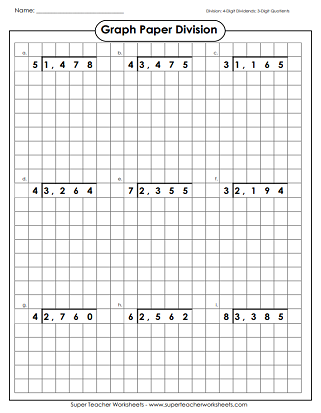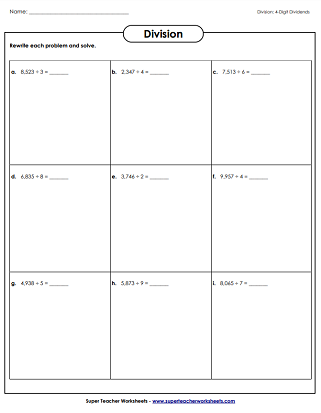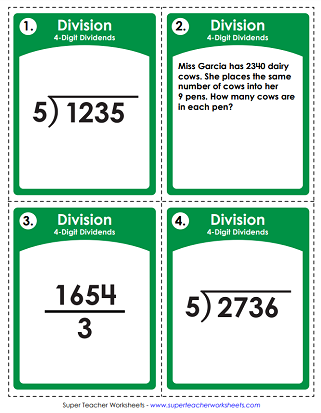# Long Division with 4-Digit Dividends

The printable worksheets and puzzles below feature long division problems with 4-digit dividends and 1-digit divisors. There's a Scoot! card game, a math crossword puzzle, and many practice worksheets.## 4-Digit Dividends

Can your students solve this math riddle: What do cats eat for breakfast? To find the answer, they'll have to complete a series of long division problems. Each problem had a dividend with four digits.
Another long division featuring 4-digit dividends, 3 or 4 digit quotients (some with remainders); Space station picture and word problem.
Long division problems with 4-digit dividends, 3 and 4 digit quotients with remainders; Whale picture and word problem.
This worksheet includes different kinds of division problems, such as word problems and a table to fill in.
This variety worksheet features different types of division questions with 4-digit dividends and remainders.
This printable file is a great math learning center activity with thirty cards to be solved by your students involving division with 4-digit dividends.
Divide problems with four-digit dividends.  The quotients are then used to complete the crossword puzzle.
Rewrite the division problems in long division form. Then solve.
This whole-class card game requires students to solve a series of long division problems with 4-digit dividends.
At the top of the page, students will find pictures of shapes with numbers in them. Follow the directions and divide. For example: Divide the numbers in the hexagons. The larger number is the dividend.
Solve the division problems with 4-digit dividends.  Place the quotients on the grid to make a superhero picture.

## Long DivisionWorksheet Generator

Division Worksheet Generator

Create your own long division worksheets! You choose the number of digits in the dividend and the divisor. Also tell if you would like remainders.

More Long Division

Choose from 2, 3, or 4-digit dividends, as well as 1 or 2-digit divisors. STW also has worksheets on dividing decimals and money.

## Sample Images ofLong Division WorksheetsMy Account
Site Information